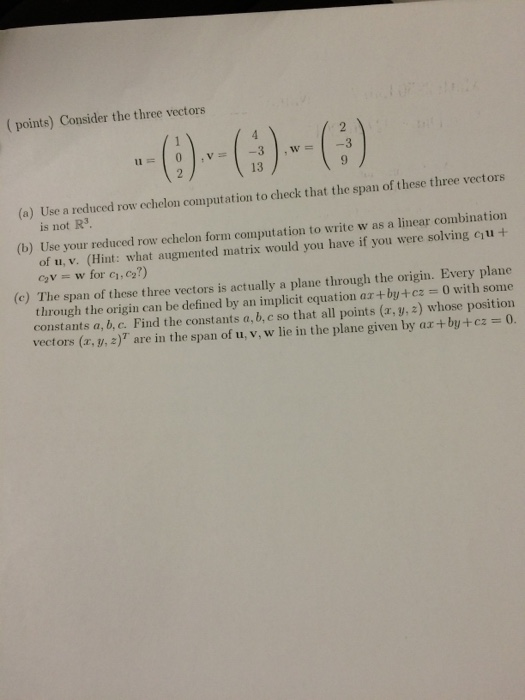# Help with this calculus vector question ? Show transcribed image text ( points) Consider the three v

Help with this calculus vector question ?Show transcribed image text ( points) Consider the three vectors (a) Use a reduced row echelon computation to check that the span of these three vectors is not R^3. (b) Use your reduced row echelon form computation to write was a linear combination of u, v. (Hint: what augmented matrix would you have if you were solving c1 u + c2v = w for c1, c2?) (c) The span of these three vectors is actually a plane through the origin. Every plane through the origin can be defined by an implicit equation ax + by + cz = 0 with sonic constants a, b. c. Find the constants a, b, c so that all points (x, y, z) whose position vectors (x, y, z)^T are in the span of u, v, w lie in the plane given by ax + by+ cz = 0.